#Quality Digest

Featured Product
This Week in Quality Digest Live
Statistics Features
Donald J. Wheeler
What do predictable processes have in common with chaos?
William A. Levinson
People can draw the wrong conclusions due to survivor, survey, and bad news bias.
Donald J. Wheeler
Does your approach do what you need?
Paul Laughlin
Correlation vs. causality
Donald J. Wheeler
In spite of what everyone says to the contrary
Statistics News
New capability delivers deeper productivity insights to help manufacturers meet labor challenges
Day and a half workshop to learn, retain, and transfer GD&T knowledge across an organization
Elsmar Cove is a leading forum for quality and standards compliance
InfinityQS’ quality solutions have helped cold food and beverage manufacturers around the world optimize quality and safety
User friendly graphical user interface makes the R-based statistical engine easily accessible to anyone
Collect measurements, visual defect information, simple Go/No-Go situations from any online device
Good quality is adding an average of 11 percent to organizations’ revenue growth
Ability to subscribe with single-user minimum, floating license, and no long-term commitment
A guide for practitioners and managersBio

Statistics

## Data Snooping, Part 1

### What pitfalls lurk within your database?

Published: Monday, August 6, 2018 - 12:03

Data mining is the foundation for the current fad of “big data.” Today’s software makes it possible to look for all kinds of relationships among the variables contained in a database. But owning a pick and shovel will not do you much good if you do not know the difference between gold and iron pyrite.

When you start rummaging around in a collection of existing data (a database) to discover if you can use some variables to “predict” other variables you are data snooping (known today as data mining). With today’s software we can go snooping in very large databases in an effort to extract useful relationships. However, in the interest of clarity we will use a small data set and do our snooping using nothing more than bivariate linear regression. The issues illustrated here are the same regardless of the size of the data set and regardless of the techniques used to “model the data.”

### Data snooping

The data set consists of five weekly production variables from a chemical plant. Figure 1 shows the data for a baseline of eight weeks of production. We will treat Y as our response variable, and see how well the other four variables do in predicting the value for Y.Figure 1: Baseline: Five variables for eight weeks of production

Figure 2 shows the results of four simple regressions using the baseline data. The relationship modeled is shown in the first column. The second column lists the coefficient of determination for each regression equation. These values explain how much of the variation in Y can be explained by the regression equation. The third column lists the p-value for the slope term of the regression equation. As always, small p-values indicate regression line slopes that are detectably different from a horizontal line.

Three of the relationships modeled have p-values that are less than the traditional 0.05. Each of these models can explain more than 80 percent of the variation in Y. The simple regression of Y = f(X4) has a p-value of 0.65, so we conclude that by itself X4 appears to be useless for predicting the value for Y.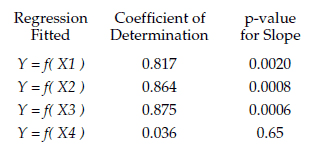Figure 2: Four simple linear regressions

### Regressions using two independent variables

In the case of the first three simple regressions listed in figure 2, we might logically ask if we can improve things by adding a second variable to our regression equation. Since the inclusion of additional variables will always increase the coefficient of determination we will need to use the conditional p-values to decide if a given addition is likely to represent a real improvement in the way our model fits the data. As always, it is the small conditional p-values that represent detectably better fits.

Figure 3 shows the results for adding a second variable to the model Y = f(X1). The bivariate regression model Y = f(X1, X2) explains 86.7 percent of the variation in the response variable Y. However, the conditional p-value for using X2 in addition to X1 is 0.21, which means that this bivariate regression model is not detectably better than Y = f(X1).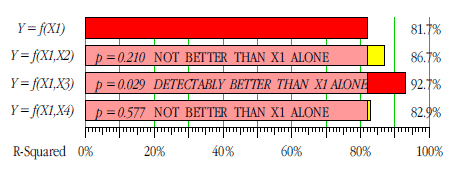Figure 3: Adding variables to Y = f(X1)

The bivariate regression model Y = f(X1, X3) explains 92.7 percent of the variation in the response variable Y. The conditional p-value for using X3 in addition to X1 is 0.029. Since this value is less than the traditional 0.05 alpha level, this bivariate regression model can be said to be detectably better than Y = f(X1).

The bivariate regression model Y = f(X1, X4) explains 82.9 percent of the variation in the response variable Y. However, the conditional p-value for using X4 in addition to X1 is 0.577, which means that this bivariate regression model is not detectably better than Y = f(X1).

Thus, out of these four models we would pick Y = f(X1, X3) as the best choice for explaining and predicting the response variable Y.

Figure 4 shows the results for adding a second variable to the model Y = f(X2). The bivariate regression model Y = f(X2, X1) explains 86.7 percent of the variation in the response variable Y. However, the conditional p-value for using X1 in addition to X2 is 0.73, which means that this bivariate regression model is not detectably better than Y = f(X2).Figure 4: Adding variables to Y = f(X2)

The bivariate regression model Y = f(X2, X3) explains 91.2 percent of the variation in the response variable Y. However, the conditional p-value for using X3 in addition to X2 is 0.143, which means that this bivariate regression model is not detectably better than Y = f(X2).

The bivariate regression model Y = f(X2, X4) explains 90.2 percent of the variation in the response variable Y. However, the conditional p-value for using X4 in addition to X2 is 0.207, which means that this bivariate regression model is not detectably better than Y = f(X2).

Thus, out of these four models we would pick Y = f(X2) as the best choice for explaining and predicting the response variable Y.

Figure 5 shows the results for adding a second variable to the model Y = f(X3). The bivariate regression model Y = f(X3, X1) explains 92.7 percent of the variation in the response variable Y. However, the conditional p-value for using X1 in addition to X3 is 0.103, which means that this bivariate regression model is not detectably better than Y = f(X3). So while figure 3 shows that adding X3 to X1 is better than using X1 alone, here we find that adding X1 to X3 is not better than using X3 alone.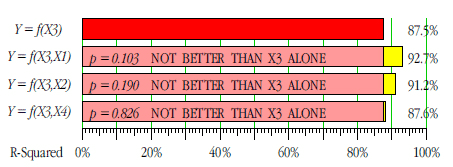Figure 5: Adding variables to Y = f(X3)

The bivariate regression model Y = f(X3, X2) explains 91.2 percent of the variation in the response variable Y. However, the conditional p-value for using X2 in addition to X3 is 0.190, which means that this bivariate regression model is not detectably better than Y = f(X3).

The regression model Y = f(X3, X4) explains 87.6 percent of the variation in the response variable Y. However, the conditional p-value for using X4 in addition to X3 is 0.826, which means that this bivariate regression model is not detectably better than Y = f(X3).

Thus, out of these 10 models our best choices for explaining and predicting the response variable Y are either Y = f(X2) or Y = f(X3). In this case, bivariate regressions do not result in detectably better fits to the baseline data. Given the extremely small size of this illustrative data set we shall not consider regressions using three or four variables.

The data for weeks 9 through 25 are shown in figure 6.Figure 6: Data for weeks 9 through 25

When we go data snooping using all 25 records of figures 1 and 6 combined we get the simple regressions of figure 7. There only two simple regressions that have a p-value less than 0.05. The regression on X3 explains about 29 percent of the variation in Y, while the regression on X4 explains 71 percent of the variation in the response variable Y. These results are considerably different from what we found earlier in figure 2.Figure 7: Four simple linear regressions for all 25 weeks

The baseline data led us to expect that a simple regression using either X2 or X3 would predict about 85 percent of the variation in the response variable Y. The combined data suggests that a simple regression using X4 will predict about 71 percent of the variation in Y. So which analysis is right?

The key to understanding what is happening here is to compare the values for X4 in figure 1 with the values for X4 in figure 6.

### What happened?

The response variable Y represents the weekly steam usage for the plant. X1 represents the amount of fatty acid in storage. X2 represents the amount of glycerin produced. X3 is the number of hours of operation for the plant. X4 is the weekly average temperature for the plant site. Since steam is used both for process heat and for heating the buildings, the amount of steam used increased during colder weeks. This was missed in the initial analysis because the baseline data came from summer weeks.

The scatterplots and regressions for the model Y = f(X4) for the baseline and combined data sets are shown in figures 8 and 9.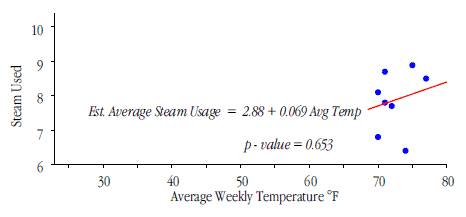Figure 8: Regression of Y upon X4 for baseline data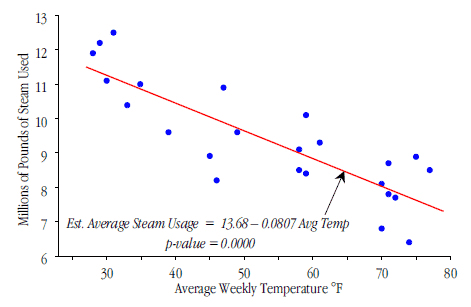Figure 9: Regression of Y upon X4 for combined data

Because the range of values for X4 was restricted in the baseline data, the first analysis missed the relationship between X4 and Y even though this relationship is the most dominant single relationship in these data. This illustrates the first caveat:

The First Caveat of Data Snooping:
Important relationships may be missed
when the data set does not contain
the full range of routine values for some variables.

This one caveat places all data snooping on a slippery slope. All of your data are historical. All of your data snooping applies to what has already happened. All of the questions of interest pertain to what will happen in the future. We collect and analyze data in order to make predictions so that we can take appropriate actions. But how can we do this when we may end up missing some important predictors?

### Data snooping out of necessity

There are situations where data snooping is the only option. For example, when studying accidents we have to use the existing data simply because it is hard to find any volunteers for experiments. I call this data snooping out of necessity.

When data snooping out of necessity we will generally have some idea that we are trying to examine. Here the objective is to discover if the database contains any evidence to support, or to refute, the idea. Whether this idea is derived from theory or is based on empirical observations, the idea will provide a framework for interpreting the results of the data snooping.

One such study that I was associated with was a study of the impact of the type of on-street parking (low-angle, high-angle, or parallel) upon mid-block traffic accidents. The data were sparse and expensive to collect. When analyzing these data I had to be very careful to take the context into account. Those comparisons that made no sense in context were interpreted as representing the noise in the data. Once we had established the noise level, we could look for comparisons that were more strongly supported by the data and which also made sense in context. When several different comparisons that were strongly supported by the data all told the same story, and when that story made sense in the context, then the combination of plausibility, replication, and strength of signal made the findings credible. The result? The hazard increases with the utilization. When fully utilized, every type of on-street parking appeared to be equally hazardous.

### Data snooping out of convenience

However, data snooping out of necessity is completely different from data snooping out of convenience. Data snooping out of convenience occurs when we rummage around in a database just because it is there and we want to see if we can find something. Working without either theory or experience as a guide we risk being led on walkabout by the missing data within the structure of the database.

In one case I was called in to rescue some data miners who had gotten lost in their database. I found the miners were using more than 100 variables to define what was, at most, a 12-dimensional vector space. In addition, more than 99.9 percent of the variable combinations were missing from the database. When dealing with such sparse and non-orthogonal data structures everything that is found has to be considered as very tentative simply because you are virtually certain to be fitting noise more often than you are fitting signals.

### Summary

While the example used here was extremely simple, the principle illustrated is real. The first caveat of data snooping is that we can miss important relationships because the data may not contain the full range of values for some variables. This is what makes data snooping so unsatisfactory as a general approach to data analysis. Yet with the advent of big data it seems that anyone with a computer thinks they are the data miner that will discover the mother lode. Let all data miners beware.

Next month we will look at three additional caveats of data snooping.

### Discuss